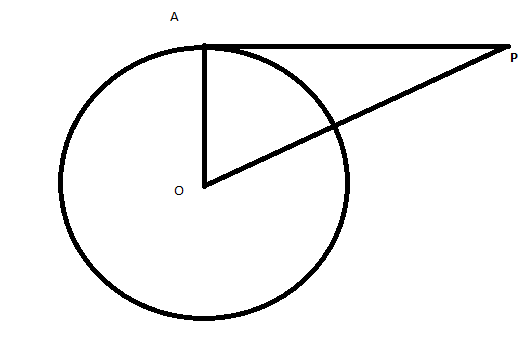Request a call back

if length of tangent from a point at distance of 5 cm from center of circle is 4 cm.find radius of the circle ?
Asked by Abhijeet abhijeet | 23 Dec, 2013, 10:25: PMExpert AnswerConsider the given figure.
Given :
Length of tangent = AP = 4 cm
Distance of point from center of circle = OP = 5cm
To Find:
Radius of the circle = OA

Property : Tangent is perpendicular to the line drawn from the center of the circle.
Thus, the triancgle OAP is right angled at A.
Applying pythagoras theorem to the triangle OAP,Thus, the radius of the circle is 3cm.
Answered by Vimala Ramamurthy | 24 Dec, 2013, 08:50: AM

## Concept Videos

CBSE 10 - Maths
in the given figure a triangle abc is drawn to circumscribe a circle of radius 3 cm such that the segment bd and dc are respectively of length 6 and 9 cm if the area of traingle abc is 54cm2 then find side ab and acAsked by jennyy | 26 Mar, 2022, 08:41: PMANSWERED BY EXPERT
CBSE 10 - Maths
Asked by sowmyashreeiyer | 31 Jan, 2022, 11:40: AMANSWERED BY EXPERT
CBSE 10 - Maths
Asked by riazu945 | 16 Jan, 2022, 01:00: PMANSWERED BY EXPERT
CBSE 10 - Maths
in the above figure majorAsked by ajabraosable27 | 11 Oct, 2021, 09:28: PMANSWERED BY EXPERT
CBSE 10 - Maths
Asked by amikasangma080 | 11 Oct, 2021, 06:14: PMANSWERED BY EXPERT
CBSE 10 - Maths
Asked by muskanmahek2411 | 08 Oct, 2021, 10:48: PMANSWERED BY EXPERT
CBSE 10 - Maths
Asked by anishasheoran372 | 13 Jul, 2021, 09:33: AMANSWERED BY EXPERT
CBSE 10 - Maths
In the adjoining figure, AB is the Diameter of the circle. E is not the centre. Angle ACD = 30°. Find angle x.Asked by bhilarevishwesh | 21 May, 2021, 08:27: AMANSWERED BY EXPERT
CBSE 10 - Maths
Q30Asked by sangyaswarupa | 16 Mar, 2021, 10:06: AMANSWERED BY EXPERT
CBSE 10 - Maths
Asked by sinhkaran79 | 14 Mar, 2021, 12:59: PMANSWERED BY EXPERT